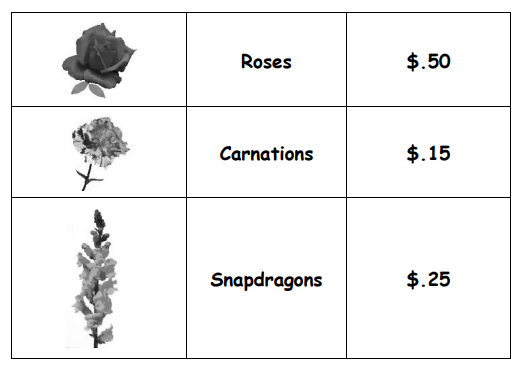Subject:
Mathematics
Problem Solving

Topics: Decimal OperationsThink BackwardsIdentify the VariablesSolve a Simpler Problem
Common Core State Standard: 2.OA.1, 2.MD.8, 4.MD.2, 5.NBT.7, 6.NS.3, 6.EE.2a, 6.EE.5, 6.EE.6, 6.EE.7, 7.EE.1, 7.EE.3, 7.EE.4a, 8.EE.7a, A-CED.1,
Knowledge and Skills:

· Can choose the correct operations in solving a problem
· Can apply the problem-solving strategy “think backwards”
· Can apply the problem-solving strategy “identify the variables”
· Can explain the reasoning used to solve a problem
· Can evaluate whether an answer to a problem is sensible in the context of the problem
· Can add and subtract decimal numbers
· Can multiply and divide decimal numbers
· Can solve simple one-variable algebraic equations (optional)

Lesson:

Procedure: This activity can be done by students individually or in pairs.

Distribute the handout and read through the first two paragraphs with the class, asking questions to ensure that students understand the problem. (If you have a digital projector and internet connection you may wish to launch this activity by visiting florist web sites such as www.ftd.com.)

There are several approaches to the solution. Three of them are outlined below; in each case you should offer an appropriate degree of problem-solving guidance for your students.

1) (2nd-4th grade) Students choose objects (or draw and cut out pictures) to represent the roses, carnations, and snapdragons, and label them with their prices. They can then try various combinations, each time adding the decimals to find the total cost of the bouquet (or, if you wish to have them practice whole numbers addition, all prices can be converted to cents).

2) (4th-6th grade) Start with the idea of a completed bouquet, and “think backwards” to realize that if there are equal numbers of roses, carnations and snapdragons in the bouquet, then
you could break it up into several small bouquets, each with 1 rose, 1 carnation, and 1 snapdragon. Add to find the cost of that bouquet, and use that information to determine how many bouquets could be combined for a total cost of around \$6.00 (the three-flower bouquet costs \$.90, divide this into \$6.00 to determine that the large bouquet could consist of 6 or 7 three-flower bouquets, a total of 18-21 flowers).

3) (7th-9th grade) Use the variable r to represent the number of roses in the bouquet. Because there will be an equal number of the carnations and snapdragons, r also represents the quantity of each of those flowers. This gives the equation

.50r + .25r + .15r = 6

(Or, if you work in cents instead of dollars:

50r + 25r + 15r = 600)

Solve the equation to find r. This will have a decimal fraction part, which doesn’t make sense in the context of the problem, so you would round it up or down to a whole number to find the quantity of each flower.

Once students have solved this problem, no matter the approach, ask them to write a clear explanation of how they did so (individually). Then ask them to create and solve (or exchange and solve) their own similar problems. A simple variation is to change the numbers involved; a more sophisticated—and fun—variation is to change the relative quantities of flowers (e.g., the arrangement is to have twice as many carnations as roses and snapdragons).

### A Valentine’s Bouquet

You’re a florist, and you want to create a special bouquet of flowers for Valentine’s Day. You want to sell the bouquet for around \$12.00, with an arrangement of flowers that costs you
around \$6.00.

The flowers you have decided to use are roses, carnations, and snapdragons. This table shows how much you would pay for each type of flower:Suppose you decide that you want the same quantity of each flower. How many of each would you use in the arrangement?